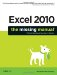Section 9.1. The World of Finance

9.1. The World of Finance

Before you start using Excel's financial functions, it helps to understand the financial concepts that lie at the heart of many of these operations. Here are some terms that those wacky accountants love to use:

• Present Value (PV) . The value of an investment or loan at the very beginning of its life. (Hopefully, after this point, the investment will rise or you'll begin to pay off the loan.) This number is also known as the principal .

• Future Value (FV) . The value of an investment or loan at some point in the future.

• Rate . The rate at which an investment or loan will increase or decrease. For example, a typical investment might have an annual interest rate of 5%, which means that after one year, the future value will be 5% larger than the present value.

• Payment (PMT) . An amount of money that's being contributed to an investment or loan. It's a regular contribution that's usually made at the same time the interest is calculated.

• Number of Payment Periods (NPER) . The total number of payment periods between the present value and the future value of an investment or loan. For example, if you've got a three-year car loan (with payments due monthly) the NPER equals 36in other words, there are 12 payment periods each year, for three years .

Usually, you'll use one of Excel's financial formulas when you have some of the information from the list above, but not all of it. For example, you might want to discover how much an investment will increase in value over a set amount of time. Or, you might want to determine what rate of savings you need to maintain in order to collect a certain amount of money for retirement.

 UP TO SPEED Knowing When to Use Positive and Negative Numbers Since Excel lets you use the same functions whether you're calculating investments or loans, the big thing to rememberand one of the hardestis whether to use positive or negative numbers. The rules of thumb are as follows : If it's money that's leaving your hands, whether it's a deposit to an account or a payment for a loan, the number should be negative. If it's money that's coming to you, whether you're receiving a loan or an investment that's matured, the number should be positive. Be on the lookout for numbers bearing an incorrect sign. If the results of your formula indicate an unusually large monthly payment, a shrinking investment, or a growing loan balance, numbers with incorrect signs may be at fault. The easiest way to test for this problem is to slightly modify your formula and see how it changes. For example, if you decrease the amount of money you want to borrow and the monthly payment increases , one of your numbers probably has the wrong sign.Excel 2010: The Missing Manual
ISBN: 1449382355
EAN: 2147483647
Year: 2003
Pages: 185

Similar book on Amazon